Teachoo Questions

Class 10
Chapter 10 Class 10 - Light - Reflection and Refraction

The password to open the file is -    teachooisbest

## Why does a light ray incident on a rectangular glass slab immersed in any medium emerges parallel to itself? Explain using a diagram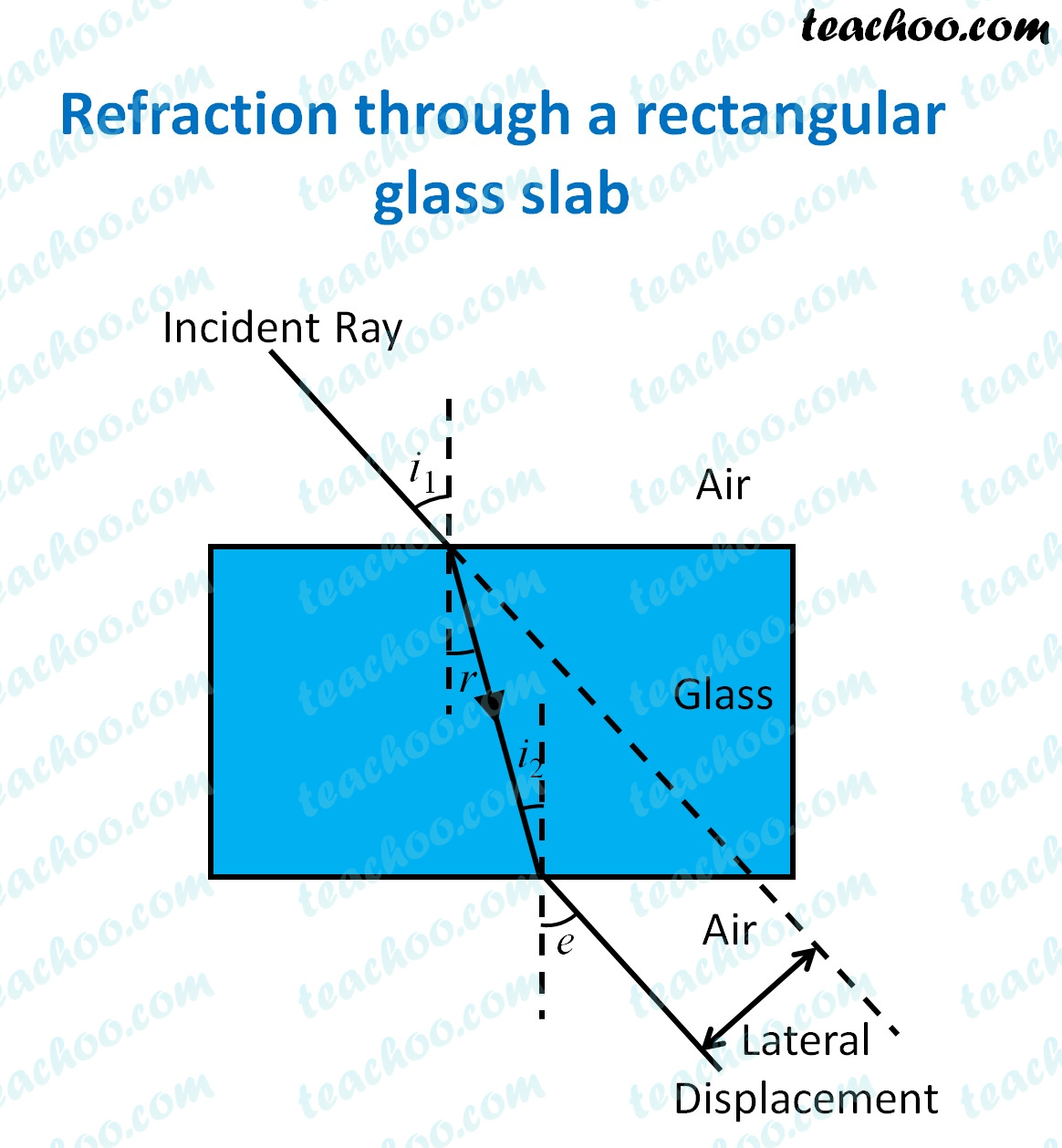When a light ray enters a glass slab,

it enters from air(rarer medium) to glass(denser medium).

As light travels from rarer to denser medium,

the ray of light moves towards the normal

When the ray of light reaches the end of the glass slab,

it emerges from glass(denser medium) to air(rarer medium).

As light travels from denser to rarer medium,

the ray of light moves away from the normal

The ray of light emerges parallel to itself because

the bending of the ray of light on top face AB (air-glass interface)

is equal and opposite to

the bending of the ray of light on bottom face CD (glass-air interface)

So, the ray of light emerges parallel to itself

## Refractive index of diamond with respect to glass is 1.6 and absolute refractive index of glass is 1.5. Find out the absolute refractive index of diamond.

Given,

Refractive index of diamond with respect to glass = 1.6

Refractive index of glass =1.5

We have to find the Refractive Index of diamond

Now,

Refractive index of diamond w.r.t glass = Refractive Index of Diamond/Refractive Index of Glass

1.6 = Refractive Index of Diamond/1.5

1.6 × 1.5 = Refractive Index of Diamond

Refractive Index of Diamond = 1.6 × 1.5

= (16/ 10) × (15/10)

= 240 / 100

= 2.4

Therefore, Refractive index of diamond is 2.4.

## Draw a ray diagram showing the path of rays of light when it enters with oblique incidence

1. from air into water;
2. from water into air

(i) from air into water

When a ray of light travels from air to water

It travels from rarer medium (air) to denser medium (water)

Hence the ray of light bends towards the normal as it enters water.

Therefore,

Angle of refraction < Angle of incidence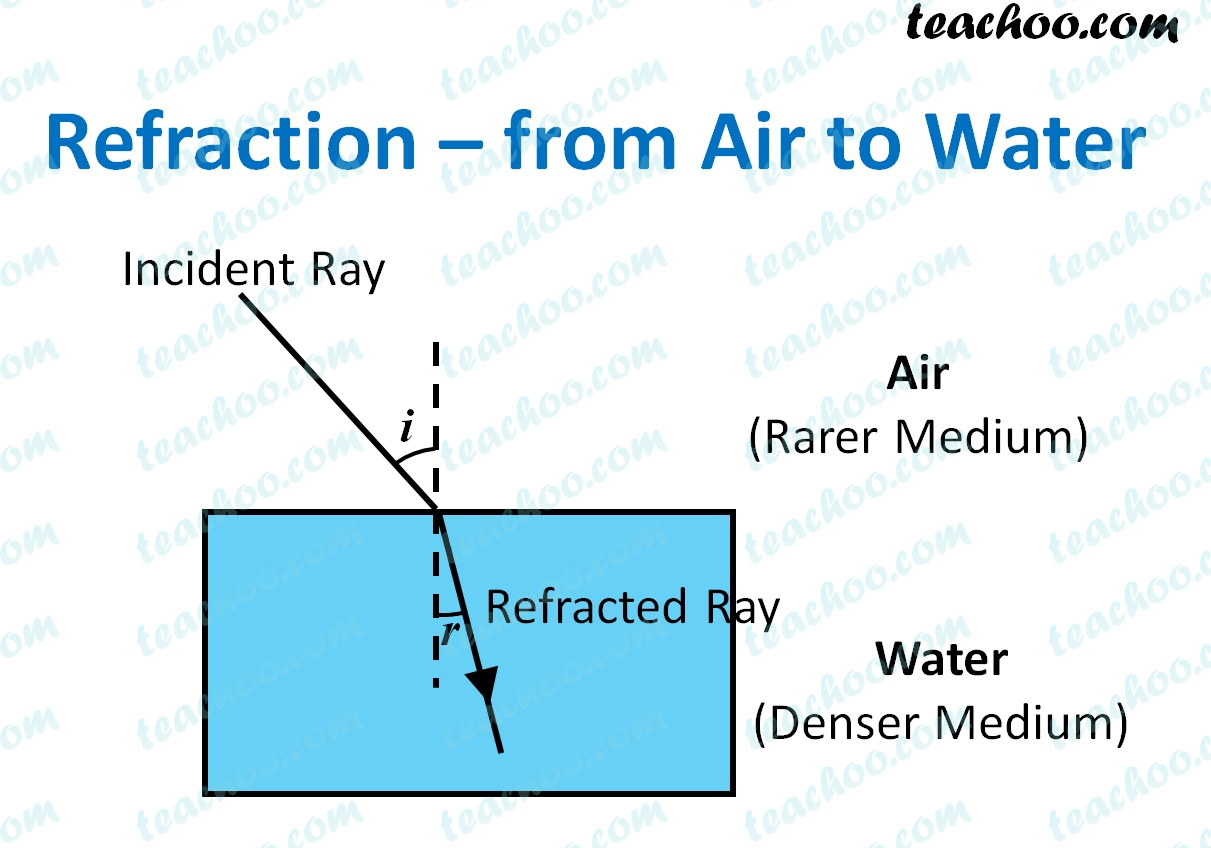(ii) from water into air

When a ray of light travels from water to air

It travels from denser medium (water) to rarer medium (air)

Hence the ray of light bends away from the normal as it enters air.

Therefore,

Angle of refraction < Angle of incidence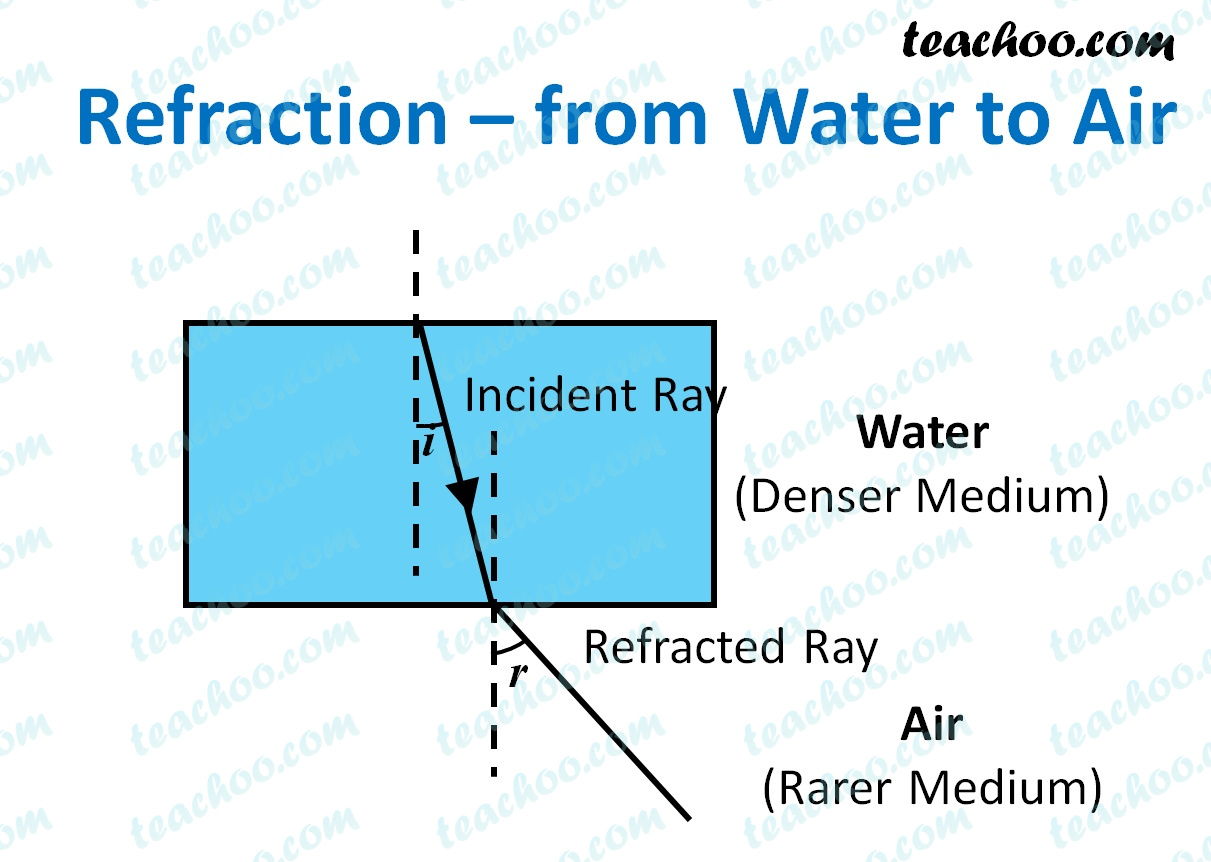## A concave mirror produces three times magnified (enlarged) real image of an object placed 10cm in front of it. Where is image located?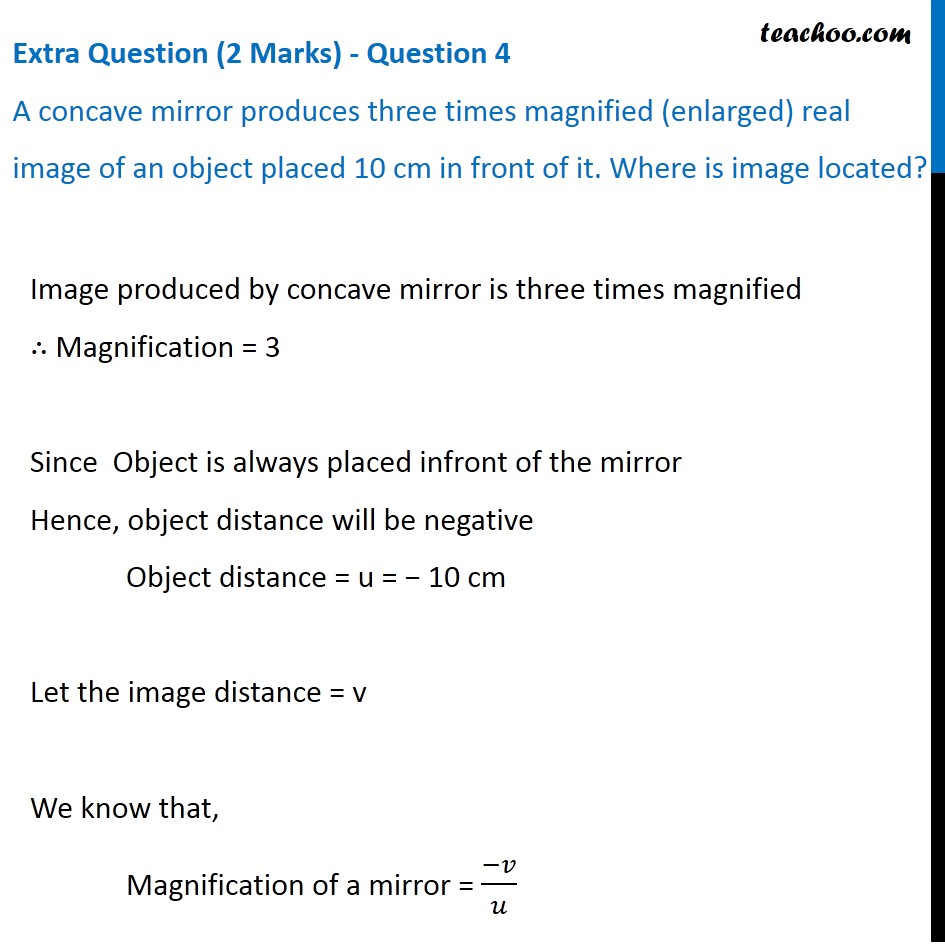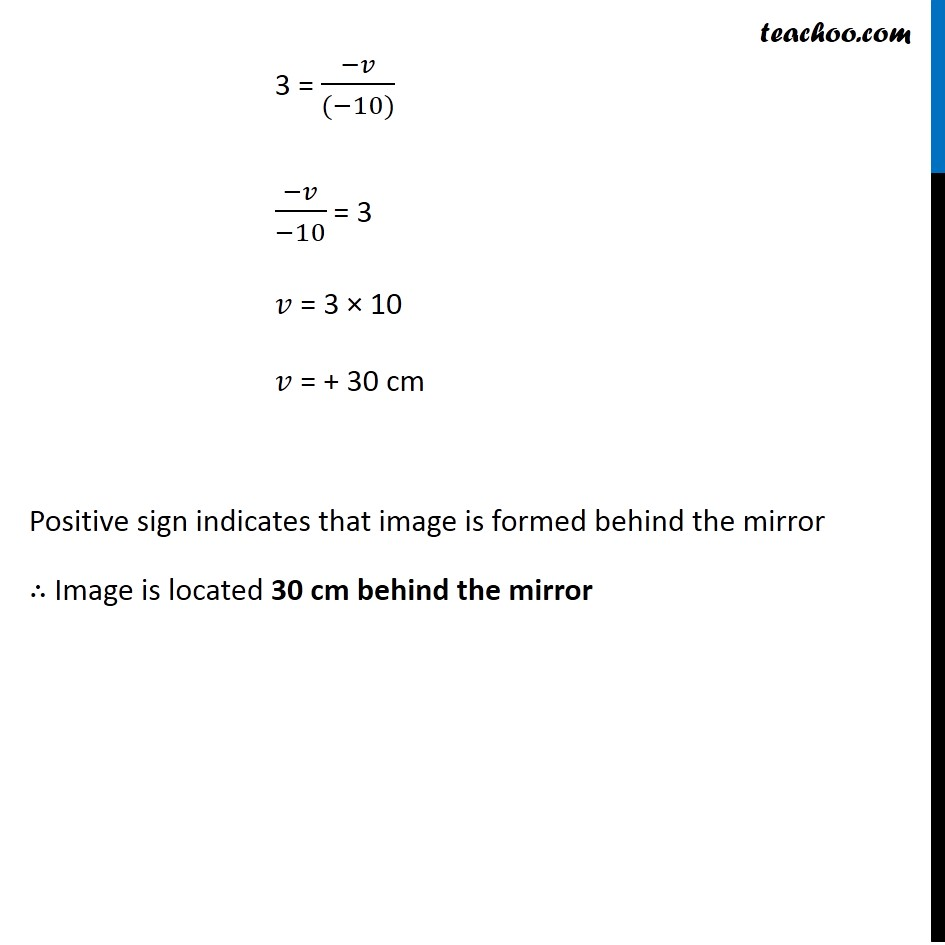## Which phenomenon occurs when light falls on

(a) highly polished surface

(b) a transparent medium

(a) highly polished surface

When a ray of light falls on a highly polished surface, reflection of light takes place.

(b) a transparent medium

When a ray of light falls on a transparent medium, refraction of light takes place.

Learn in your speed, with individual attention - Teachoo Maths 1-on-1 Class

### Transcript

Extra Question (2 Marks) - Question 4 A concave mirror produces three times magnified (enlarged) real image of an object placed 10 cm in front of it. Where is image located? Image produced by concave mirror is three times magnified ∴ Magnification = 3 Since Object is always placed infront of the mirror Hence, object distance will be negative Object distance = u = − 10 cm Let the image distance = v We know that, Magnification of a mirror = (−𝑣)/𝑢 3 = (−𝑣)/((−10)) (−𝑣)/(−10) = 3 𝑣 = 3 × 10 𝑣 = + 30 cm Positive sign indicates that image is formed behind the mirror ∴ Image is located 30 cm behind the mirror